﻿ Calculation of combustion in the engine cylinder - XSim

# Calculation of combustion in the engine cylinder

Update: January 30, 2018
OpenFOAM 4.x

## Case directory

\$FOAM_TUTORIALS/combustion/engineFoam/kivaTest

## Summary

We calculates the combustion in a piston engine cylinder operating the crank angle from -180° to 60°. The mesh file is prepared in KIVA format and we convert it to OpenFOAM format for using. The model geometry consists of three regions: cylinderHead (green), liner (red), and piston (white) as shown in the figure below.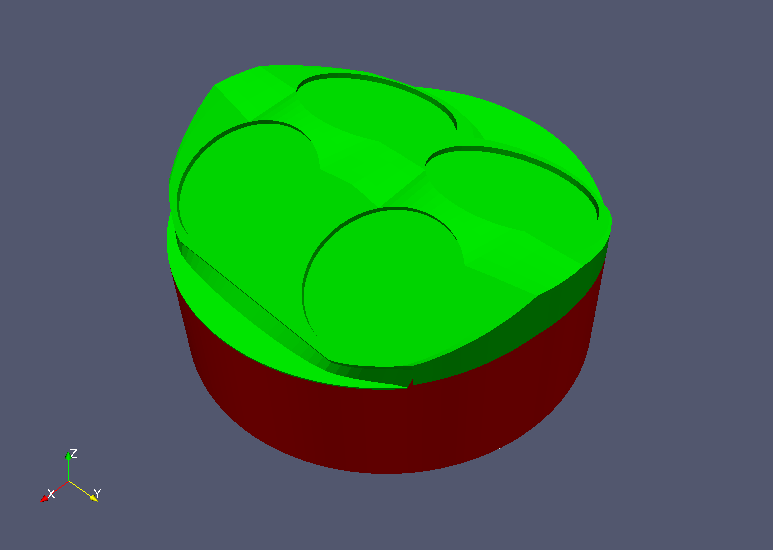Model geometry (upper)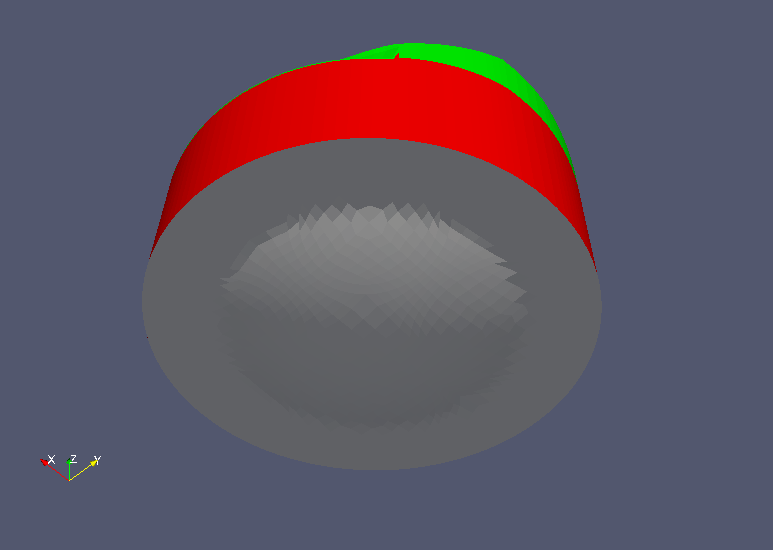Model geometry (lower)

The engine specifications are defined in the file "constant/engineGeometry" as follows.

```engineMesh      layered;
conRodLength    conRodLength [0 1 0 0 0 0 0] 0.147;
bore            bore [0 1 0 0 0 0 0] 0.092;
stroke          stroke [0 1 0 0 0 0 0] 0.08423;
clearance       clearance [0 1 0 0 0 0 0] 0.00115;
rpm             rpm [0 0 -1 0 0 0 0] 1500;
```

The settings, ignition position, etc. of the equations for combustion reaction are defined in the file "constant/combustionProperties", and the fuel, oxidant, and products of combustion are defined in the file "constant/thermophysicalProperties" as "fuel", "oxidant", and "burntProducts", respectively.

The meshes are as follows, and the number of mesh is 27544.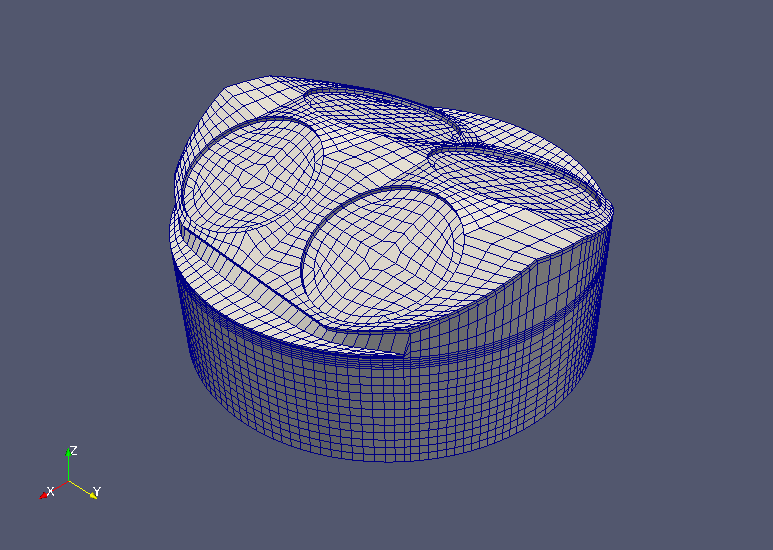Meshes (upper)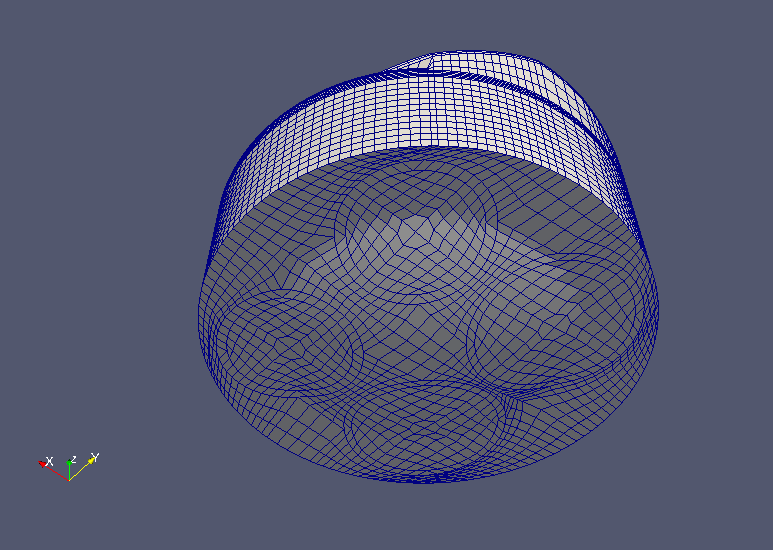Meshes (lower)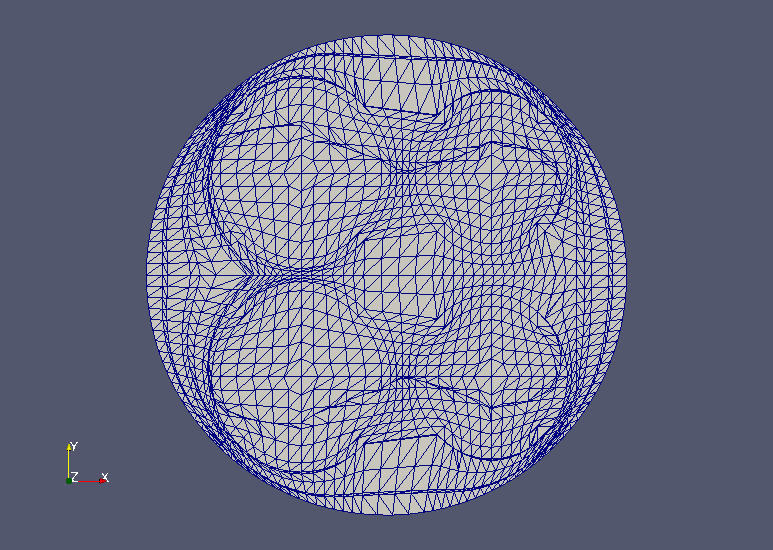Meshes (XY-plane)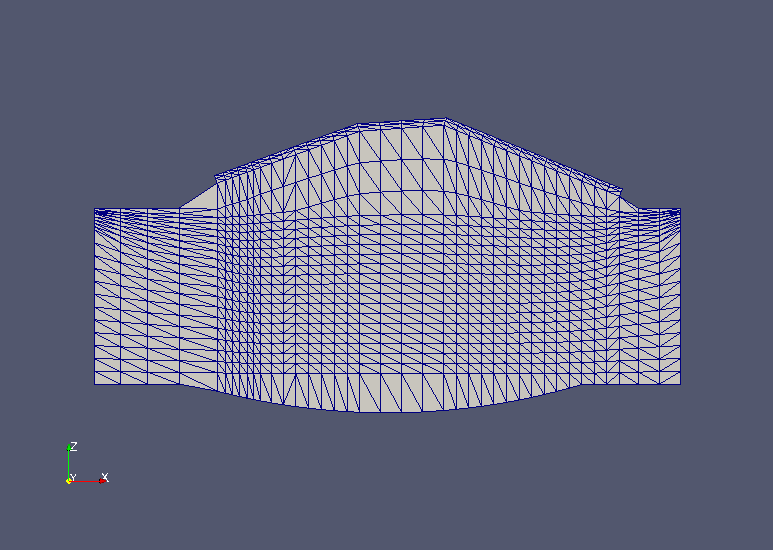Meshes (XZ-plane)

The calculation result is as follows.

Temperature distribution in the engine cylinder (T, volume rendering)

The flow velocity, pressure, and temperature distributions at a crank angle of 60° in the XY plane (Z=0.07) and XZ plane (Y=0) are shown below. A sphere in the figure indicates the ignition position.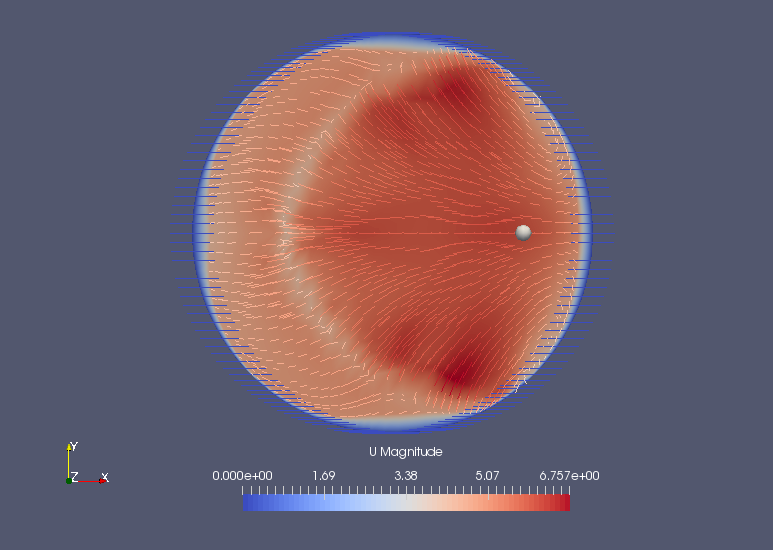Flow velocity at Z=0.07 plane (U)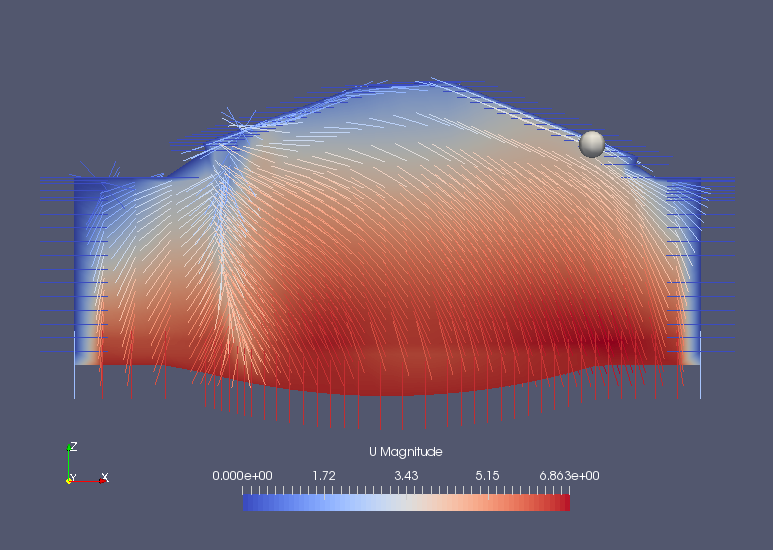Flow velocity at Y=0 plane (U)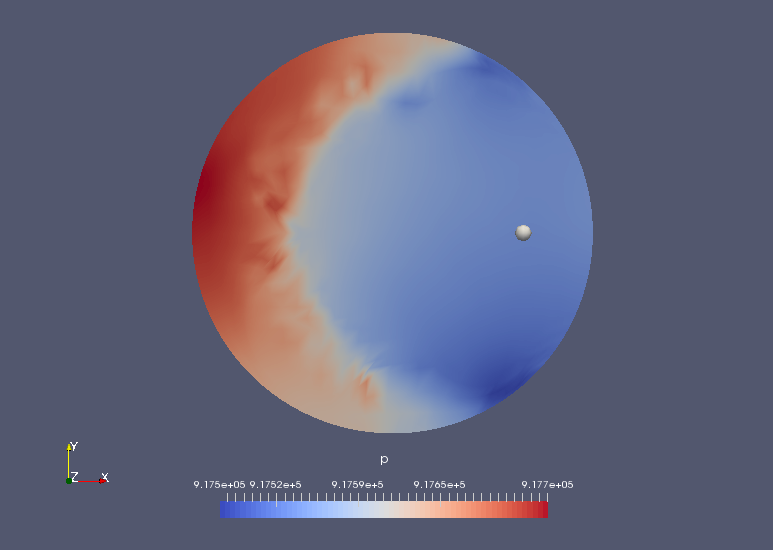Pressure at Z=0.07 plane (p)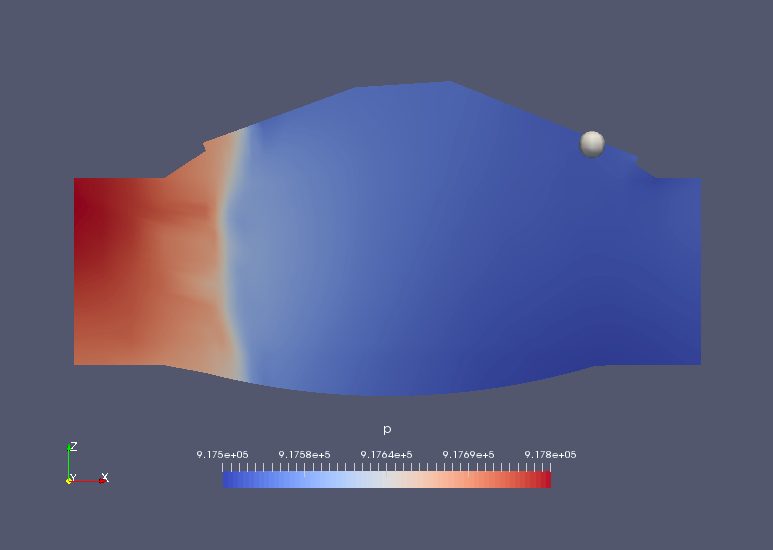Pressure at Y=0 plane (p)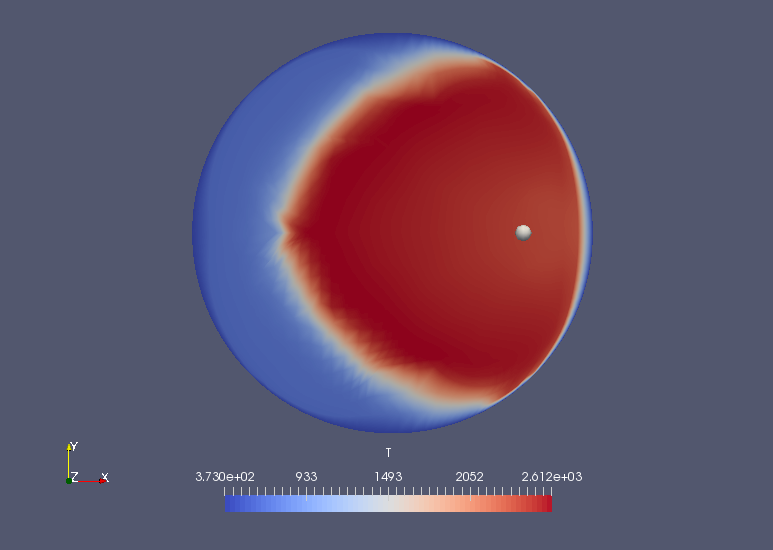Temperature at Z=0.07 plane (T)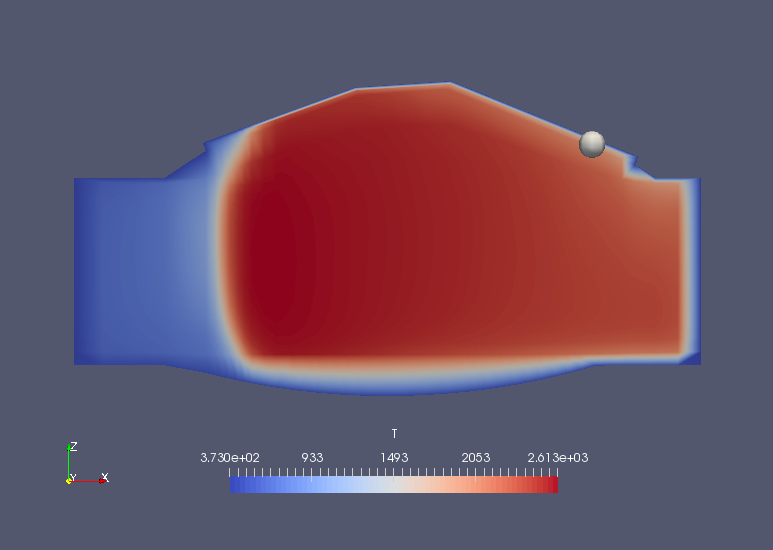Temperature at Y=0 plane (T)

And the average pressure and temperature at each crank angle are output to The file "logSummary.-180.dat".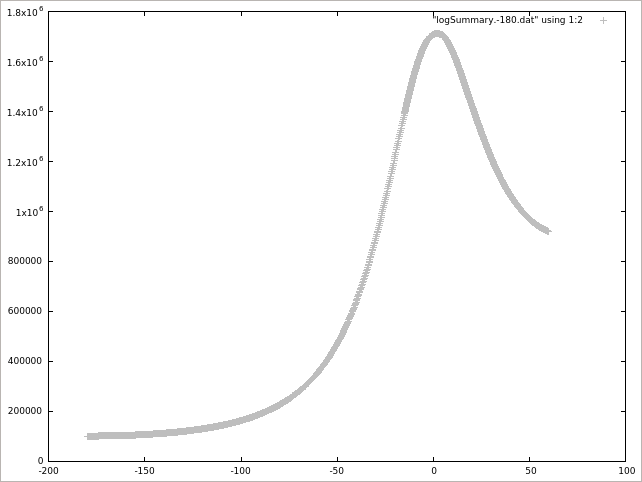Average pressure (p)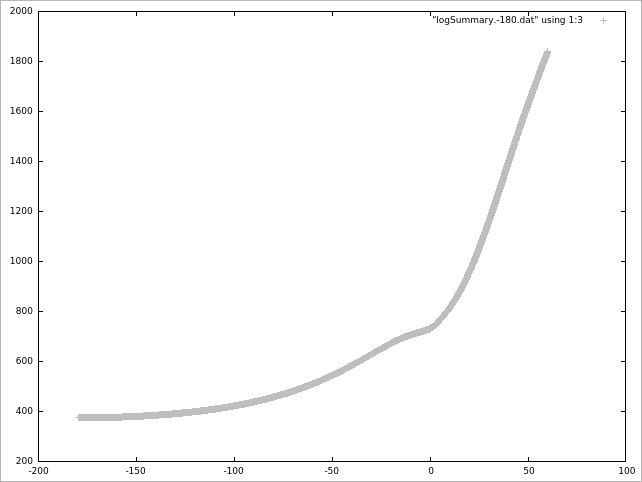Average temperature (T)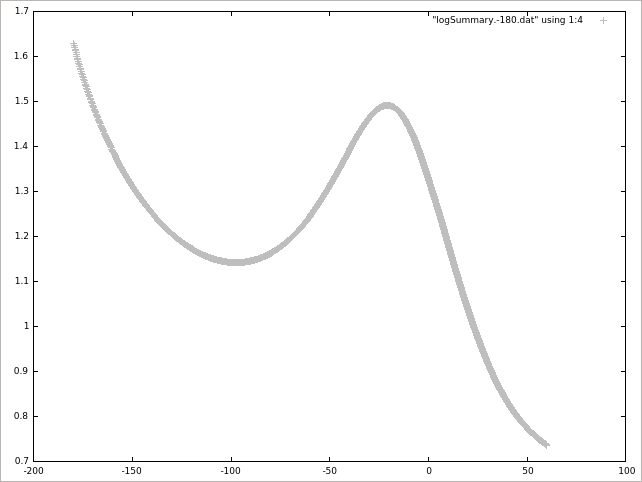Average of (k * 2/3)1/2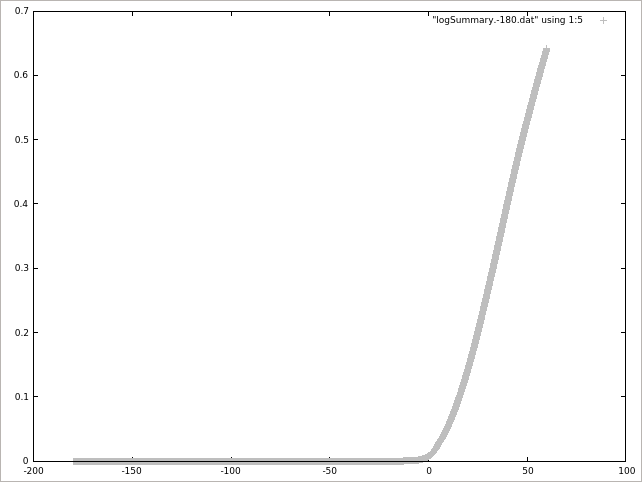1 - (Average of b)

## Commands

cp -r \$FOAM_TUTORIALS/combustion/engineFoam/kivaTest kivaTest
cd kivaTest

kivaToFoam -file otape17
engineFoam

paraFoam

gnuplot
gnuplot>plot "logSummary.-180.dat" using 1:2 lc rgb "gray"
gnuplot>plot "logSummary.-180.dat" using 1:3 lc rgb "gray"
gnuplot>plot "logSummary.-180.dat" using 1:4 lc rgb "gray"
gnuplot>plot "logSummary.-180.dat" using 1:5 lc rgb "gray"

We use the utility "kivaToFoam" to convert the KIVA format (KIVA-3V format) mesh file "otape17" into OpenFOAM format.

## Calculation time

21 minutes 33.99 seconds *Single, Inter(R) Core(TM) i7-2600 CPU @ 3.40GHz 3.40GHz